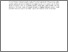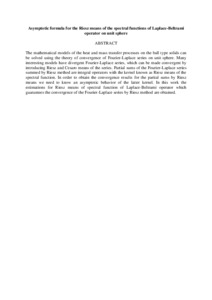# Asymptotic formula for the Riesz means of the spectral functions of Laplace-Beltrami operator on unit sphere

## Citation

Rasedee, Ahmad Fadly Nurullah and Ahatjonovich, Anvarjon Ahmedov and Abdul Sathar, Mohammad Hasan (2017) Asymptotic formula for the Riesz means of the spectral functions of Laplace-Beltrami operator on unit sphere. Journal of Physics: Conference Series, 890 (1). pp. 1-7. ISSN 1742-6588; ESSN: 1742-6596

## Abstract

The mathematical models of the heat and mass transfer processes on the ball type solids can be solved using the theory of convergence of Fourier-Laplace series on unit sphere. Many interesting models have divergent Fourier-Laplace series, which can be made convergent by introducing Riesz and Cesaro means of the series. Partial sums of the Fourier-Laplace series summed by Riesz method are integral operators with the kernel known as Riesz means of the spectral function. In order to obtain the convergence results for the partial sums by Riesz means we need to know an asymptotic behavior of the latter kernel. In this work the estimations for Riesz means of spectral function of Laplace-Beltrami operator which guarantees the convergence of the Fourier-Laplace series by Riesz method are obtained.Preview
Text (Abstract)
Asymptotic formula for the Riesz means of the spectral functions of Laplace-Beltrami operator on unit sphere.pdfView Item